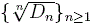Infinitely Log-monotonic Combinatorial Sequences

William Y.C. Chen, Jeremy J.F. Guo and Larry X.W. Wang

 Abstract:   We introduce the notion of infinitely log-monotonic sequences. By establishing a connection between completely monotonic functions and infinitely log-monotonic sequences, we show that the sequences of the Bernoulli numbers, the Catalan numbers and the central binomial coefficients are infinitely log-monotonic. In particular, if a sequence {an}n≥0 is log-monotonic of order two, then it is ratio log-concave in the sense that the sequence {an+1/an}n≥0 is log-concave. Furthermore, we prove that if a sequence {an}n≥k is ratio log-concave, then the sequenceis strictly logconcave subject to a certain initial condition. As consequences, we show that the sequences of the derangement numbers, the Motzkin numbers, the Fine numbers, the central Delannoy numbers, the numbers of tree-like polyhexes and the Domb numbers are ratio log-concave. For the case of the Domb numbers Dn, we confirm a conjecture of Sun on the log-concavity of the sequence.   AMS Classification:  05A20, 11B68   Keywords:   logarithmically completely monotonic function, infinitely log-monotonic sequence, ratio log-concave, Riemann zeta function   Download:   pdf

Return